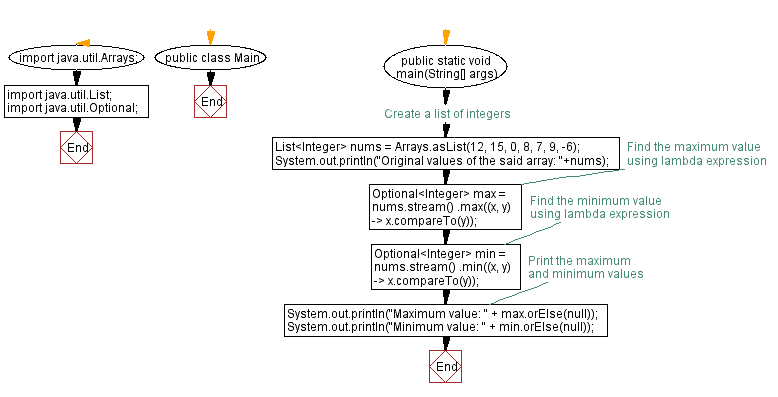# Find maximum and minimum values with Lambda expression in Java

## Java Lambda Program: Exercise-11 with Solution

Write a Java program to implement a lambda expression to find the maximum and minimum values in a list of integers.

Sample Solution:

Java Code:

``````import java.util.Arrays;
import java.util.List;
import java.util.Optional;

public class Main {
public static void main(String[] args) {
// Create a list of integers
List<Integer> nums = Arrays.asList(12, 15, 0, 8, 7, 9, -6);
System.out.println("Original values of the said array: "+nums);
// Find the maximum value using lambda expression
Optional<Integer> max = nums.stream()
.max((x, y) -> x.compareTo(y));

// Find the minimum value using lambda expression
Optional<Integer> min = nums.stream()
.min((x, y) -> x.compareTo(y));

// Print the maximum and minimum values
System.out.println("Maximum value: " + max.orElse(null));
System.out.println("Minimum value: " + min.orElse(null));
}
}
``````

Sample Output:

```Original values of the said array: [12, 15, 0, 8, 7, 9, -6]
Maximum value: 15
Minimum value: -6
```

Explanation:

The first step is to import the necessary classes: Arrays, Lists, and Optionals.

Using Arrays.asList(), we create a list of integers named nums in the main method. The list contains several integer values.

To find the maximum value, we use the stream() method on the nums list to convert it into a stream. Then, we call the max() method on the stream and provide a lambda expression (x, y) -> x.compareTo(y) as a comparator. This lambda expression compares two integers x and y using the compareTo() method. This method returns a negative, zero, or positive value depending on the comparison result.

The max() method returns an Optional object that may contain the maximum value if it exists or an empty Optional if the stream is empty.

Similarly, we use the min() method on the stream with the same lambda expression to find the minimum value.

The max() and min() methods return Optional objects that may contain the maximum and minimum values, respectively.

Finally, we use the orElse() method on the Optional objects to retrieve the maximum and minimum values. If the Optional object is empty, we display null.

Lastly, we print the maximum and minimum values using System.out.println ().

Flowchart:Live Demo:

Java Code Editor:

Improve this sample solution and post your code through Disqus

Java Lambda Exercises Previous: Concatenate strings using Lambda expression in Java.
Java Lambda Exercises Next: Multiply and sum elements with Lambda expression in Java.

What is the difficulty level of this exercise?

Test your Programming skills with w3resource's quiz.

﻿